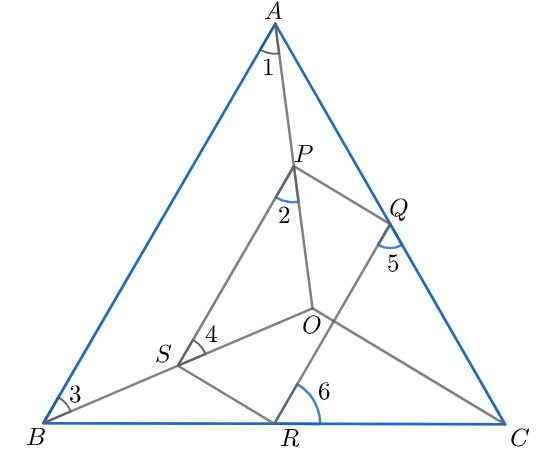Courses
Courses for Kids
Free study material
Offline Centres
MoreLast updated date: 04th Dec 2023
Total views: 282k
Views today: 2.82k

# In the given figure, if PQRS is a parallelogram and $AB||PS$ , then prove that $OC||SR$ .Verified
282k+ views
Hint: Problems related to triangles and parallelograms are a bit tricky to understand, but can be solved very easily if we get all the key concepts behind the problem. We need to be fluent with some basic as well as some advanced topics of triangles, parallelograms, similarity of triangles and their various properties. A parallelogram is a closed bounded figure consisting of four sides, in which opposite sides are equal and are parallel to one another. The lines which are marked with an arrow, are parallel to one another.

Now we start off with the solution to the given problem. In the triangles $OAB\text{ and }OPS$ , we have $AB||PS$ and $\angle 1=\angle 2$ and $\angle 3=\angle 4$ . Thus we can say triangles $OAB\text{ and }OPS$ are similar. We can write,
$\dfrac{OP}{OA}=\dfrac{OS}{OB}=\dfrac{PS}{AB}.......\left( i \right)$
We have $AB||PS||QR$ . Now in triangles $CQR\text{ and }CAB$ , we have $AB||QR$ and $\angle 1=\angle 2$ and $\angle 3=\angle 4$ . Now we can say that the triangles $CQR\text{ and }CAB$ are similar to one another. We can then write,
$\dfrac{CQ}{CA}=\dfrac{CR}{CB}=\dfrac{QR}{AB}.......\left( ii \right)$
$\dfrac{PS}{AB}=\dfrac{CR}{CB}=\dfrac{CQ}{CA}.......\left( iii \right)$
$\Rightarrow \dfrac{CR}{CB}=\dfrac{OS}{OB}.......\left( iv \right)$ from the equations (i) and (iii)
From the above relation that we have, we can say this equation holds good for triangles $BOC$ . Now from the intermediate equation we can say that $OC||SR$ .